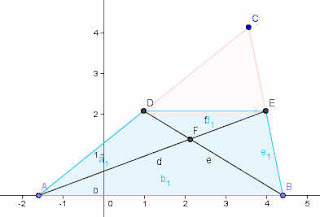## Wednesday, 27 January 2010

### Geometric Explorations in Three Stages

Here are some geometry explorations I think that you could present to kids in three levels.. For example:

If you had a triangle and marked one of the points of concurrency, perhaps the intersection of the medians or the intersection of the perpendicular bisectors.

First you challenge the student to think about what would happen if??? What if we held two of the vertices fixed, and moved the third along a line parallel to the side joining the first two; what would be the path traced out by the int

It is good for them to ponder the "what might happen" without a definite goal or a right or wrong answer. Maybe doodle with a pencil and paper... Push for a little detail.

Now (this would be stage two) let them actually construct the locus with interactive software and see what happens...this is not trivial, even with the magic of modern software like Geogebra or Cabri or Geometer's Sketchpad... and there are often surprises that lead them to wonder their own "what if" questions.

Now the final stage... prove it analytically. This will be the hardest part. For example, If you picked the centroid, or intersection of the perpendicular bisectors of the sides, then what they will see is that the locus it follows is a straight line parallel to the fixed side; but you can be a little more definite.. Let's call the two fixed points A and B, and put them on the x-axis. Now the third point will have a changing value of x, but the y-coordinate will be constant at some value, call it C. If they have trouble starting, we can ask them to think what is the coordinates of the point M that is the median of AC, and N that is the median of BC..... again the x values will change, but the y-values will be C/2 for both. So now we can challenge them to describe the geometric figure that is AMNB, whose diagonals are the medians, and whose intersection we seek.So what can we see in the trapezoid??... what do we know??... what about the size of the two parallel bases??... Do you see any triangle relations that would help? Eventually we hope they will figure out that triangles AFB and EFD are similar.. and since the two bases are in a ratio of one to two, the perpendicular distance to the centroid will be in that same ratio... or 2/3 of the way from the lower base to the upper base.... SO??? aha, but the upper base of the trapezoid has a y-coordinate of C/2, so 2/3 of that means the centroid will be at C/3...

For an advanced challenge, what happens if we follow the intersection of the angle bisectors, or the intersection of the altitudes, the locus will not be a straight line. Can you visualize it? Construct it.. what does it look like... Prove it?